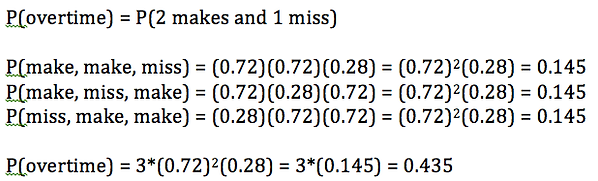top of page

## Chapter 6 - Day 6

##### Learning Targets
• Determine whether the conditions for a binomial setting are met.

• Calculate and interpret probabilities involving binomial distributions

##### Activity: Is it Smart to Foul at the End of the Game?

This activity is no longer available at Mathalicious, but the full game is available on YouTube. Here are links to the important parts of the game needed for this lesson. Be sure to review the lesson before teaching it so you are ready to pause the video at the right time.

Setting up the free throws

First shot

Second shot

Third shotWe scaffolded students through probability calculations as we slowly revealed the outcome of each free throw. This lesson has some great connections to Chapter 5 (independent events, conditional probability), but our focus was on developing the conceptual understanding of the binomial formula.  Instead of simply giving them the binomial formula, this lesson helps students to understand the components of the binomial formula (experience first, formalize later!).

The best context for discussing the binomial formula is when calculating the probability of overtime.So what does the 3 represent?  It is the number of ways of making 2 and missing 1.  This can also be calculated using a combination (3 choose 2).  Discussing this idea with students is vital if they are to understand the binomial formula.

##### Luke's Lesson Notes

Here is a brief video highlighting some key information to help you prepare to teach this lesson.

bottom of page